## Summary

I made a tutorial about planar mapping previously. The biggest disadvantage of the technique is that it only works from one direction and breaks when the surface we’re drawing isn’t oriented towards the direction we’re mapping from (up in the previous example). A way to improve automatic uv generation is that we do the mapping three times from different directions and blend between those three colors.

This tutorial will build upon the planar mapping shader which is a unlit shader, but you can use the technique with many shaders, including surface shaders.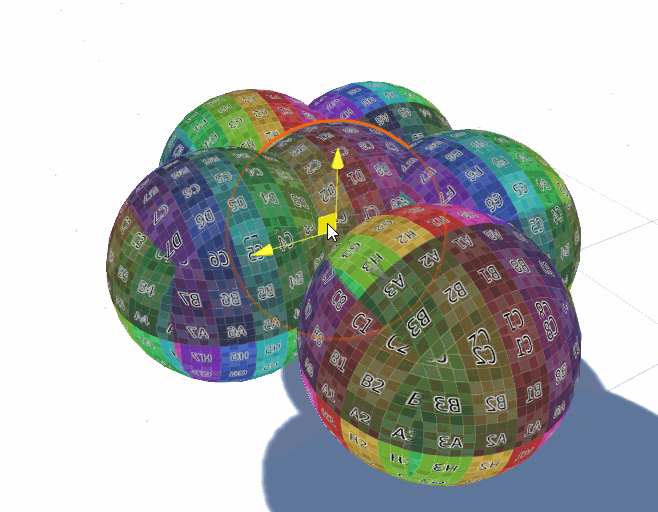## Calculate Projection Planes

To generate three different sets of UV coordinates, we start by changing the way we get the UV coordinates. Instead of returning the transformed uv coordinates from the vertex shader we return the world position and then generate the UV coordinates in the fragment shader.

``````struct v2f{
float4 position : SV_POSITION;
float3 worldPos : TEXCOORD0;
};

v2f vert(appdata v){
v2f o;
//calculate the position in clip space to render the object
o.position = UnityObjectToClipPos(v.vertex);
//calculate world position of vertex
float4 worldPos = mul(unity_ObjectToWorld, v.vertex);
o.worldPos = worldPos.xyz;
return o;
}
``````

We use transform tex to apply the tiling and offset of the texture like we’re used to. In my shader I use xy and zy so the world up axis is mapped to the y axis of the texture for both textures, not rotating them in relation to each other, but you can play around with the way use use those values (the way the top UVs are mapped is arbitrary).

``````fixed4 frag(v2f i) : SV_TARGET{
//calculate UV coordinates for three projections
float2 uv_front = TRANSFORM_TEX(i.worldPos.xy, _MainTex);
float2 uv_side = TRANSFORM_TEX(i.worldPos.zy, _MainTex);
float2 uv_top = TRANSFORM_TEX(i.worldPos.xz, _MainTex);
``````

After obtaining the correct coordinates, we read the texture at those coordinates, add the three colors and divide the result by 3 (adding three colors without dividing by the number of colors would just be very bright).

``````//read texture at uv position of the three projections
fixed4 col_front = tex2D(_MainTex, uv_front);
fixed4 col_side = tex2D(_MainTex, uv_side);
fixed4 col_top = tex2D(_MainTex, uv_top);

//combine the projected colors
fixed4 col = (col_front + col_side + col_top) / 3;

//multiply texture color with tint color
col *= _Color;
return col;
``````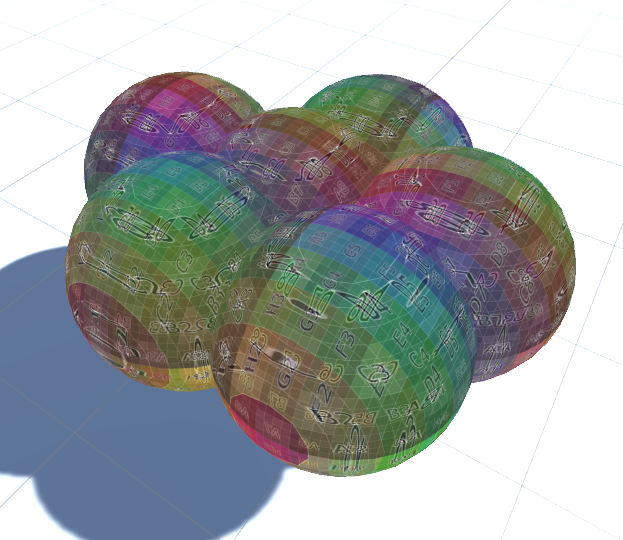## Normals

Having done that our material looks really weird. That’s because we display the average of the three projections. To fix that we have to show different projections based on the direction the surface is facing. The facing direction of the surface is also called “normal” and it’s saved in the object files, just like the position of the vertices.

So what we do is get the normals in our input struct, convert them to worldspace normals in the vertex shader (because our projection is in worldspace, if we used object space projection we’d keep the normals in object space).

For the conversion of the normal from object space to world space, we have to multiply it with the inverse transposed matrix. It’s not important to understand how that works exactly (matrix multiplication is complicated), but I’d like to explain why we can’t just multiply it with the object to world matrix like we do with the position. The normals are orthogonal to the surface, so when we scale the surface only along the X axis and not the Y axis the surface gets steeper, but when we do the same to our normal, it also points more upwards than previously and isn’t orthogonal to the surface anymore. Instead we have to make the normal more flat the steeper the surface gets and the inverse transpose matrix does that for us. Then we also convert the matrix to a 3x3 matrix, discarding the parts that would move the normals. (we don’t want to move the normals because they represent directions instead of positions)

The way we use the inverse transpose object to world matrix is that we multiply the normal with the world to object matrix (previously we multiplied the matrix with the vector, order is important here).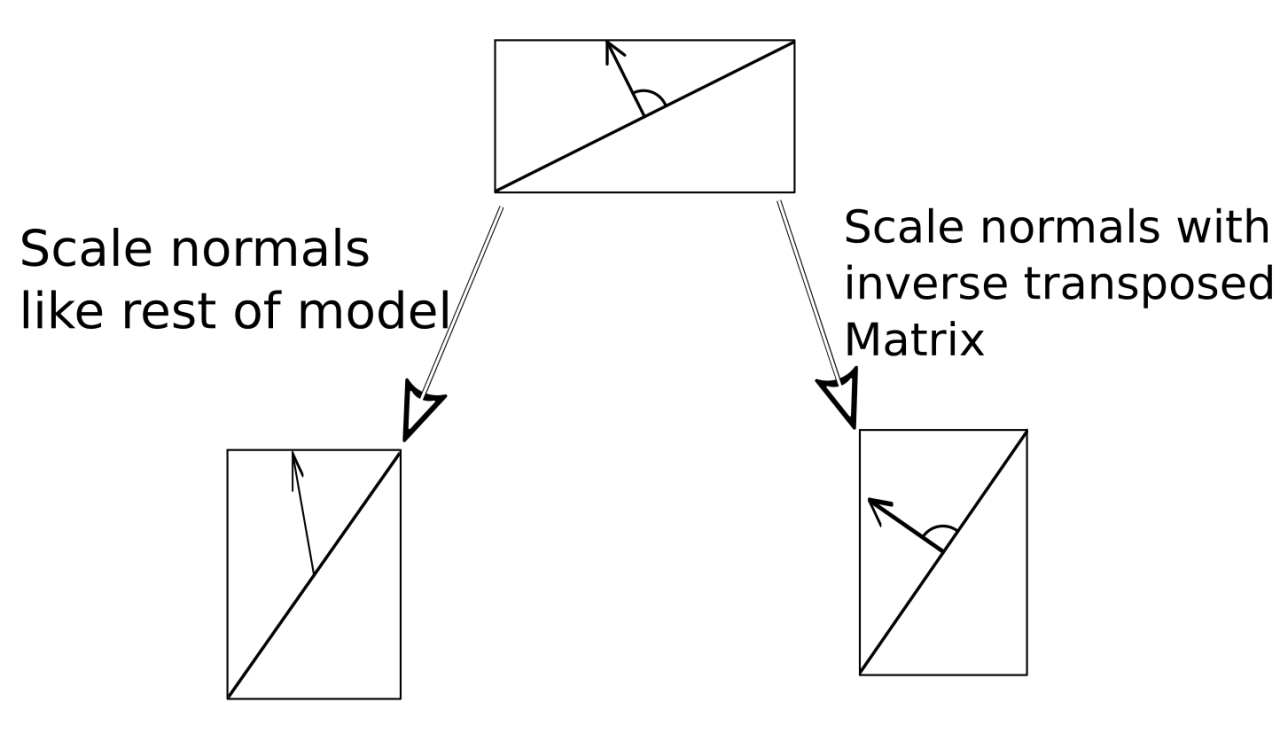``````struct appdata{
float4 vertex : POSITION;
float3 normal : NORMAL;
};

struct v2f{
float4 position : SV_POSITION;
float3 worldPos : TEXCOORD0;
float3 normal : NORMAL;
};

v2f vert(appdata v){
v2f o;
//calculate the position in clip space to render the object
o.position = UnityObjectToClipPos(v.vertex);
//calculate world position of vertex
float4 worldPos = mul(unity_ObjectToWorld, v.vertex);
o.worldPos = worldPos.xyz;
//calculate world normal
float3 worldNormal = mul(v.normal, (float3x3)unity_WorldToObject);
o.normal = normalize(worldNormal);
return o;
}
``````

To check our normals, we can now just return them in our fragment shader and see the different axis as colors.

``````fixed4 frag(v2f i) : SV_TARGET{
return fixed4(i.normal.xyz, 1);
}
``````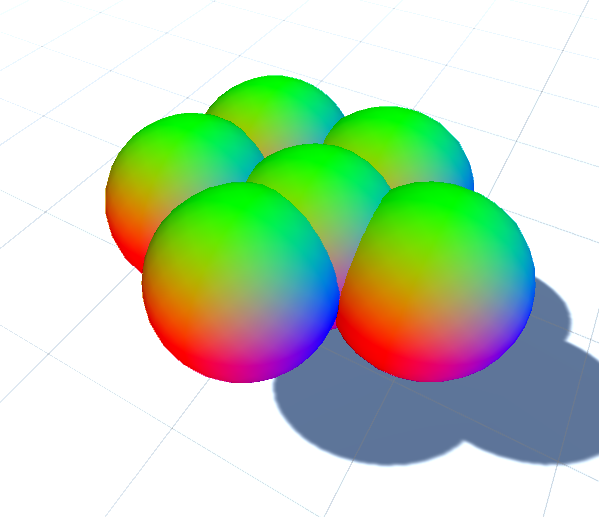To convert the normals to weights for the different projections we start by taking the absolute value of the normal. That’s because the normals go in the positive and negative directions. That’s also why in our debug view the “backside” of our object, where the axes go towards the negative direction, is black.

``````float3 weights = i.normal;
weights = abs(weights);
``````

After that we can multiply the different projections with the weights, making them only appear on the side we’re projecting it on, not the others where the texture looks stretched. We multiply the projection from the xy plane to the z weight because towards that axis it doesn’t stetch and we do a smiliar thing to the other axes.

We also remove the division by 3 because we don’t add them all together anymore.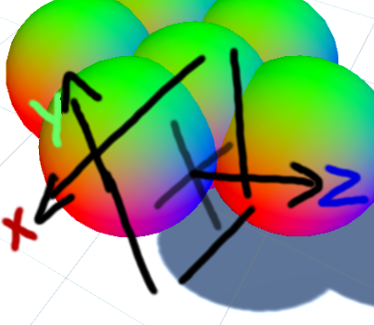``````//generate weights from world normals
float3 weights = i.normal;
//show texture on both sides of the object (positive and negative)
weights = abs(weights);

//combine weights with projected colors
col_front *= weights.z;
col_side *= weights.x;
col_top *= weights.y;

//combine the projected colors
fixed4 col = col_front + col_side + col_top;

//multiply texture color with tint color
col *= _Color;
return col;
``````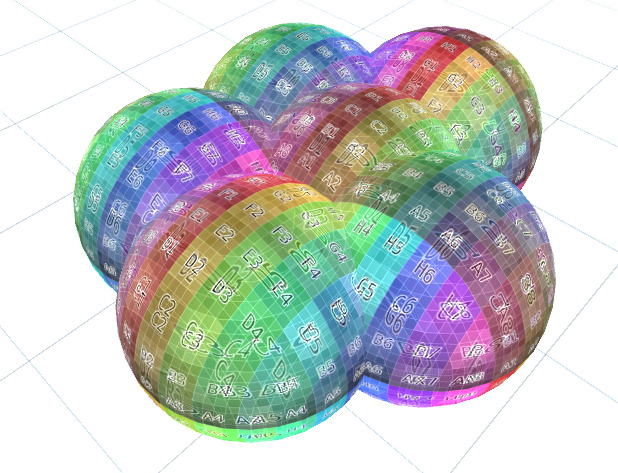That’s way better already, but now we have the same problem again why we added the division by 3, the components of the normals add up to more than 3 sometimes, making the texture appear brighter than it should be. We can fix that by dividing it by the sum of it’s components, forcing it to add up to 1.

``````//make it so the sum of all components is 1
weights = weights / (weights.x + weights.y + weights.z);
``````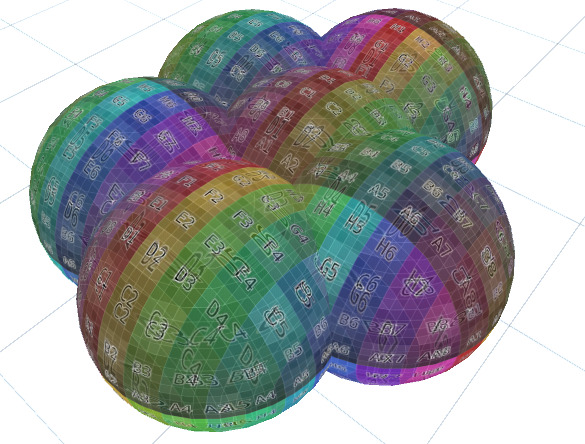And with that we’re back to the expected brightness.

The last thing we add to this shader is the possibility to make the different directions more distinct, because right now the area where they blend into each other is still pretty big, making the colors look messy. To archieve that we add a new property for the sharpness of the blending. Then, before making the weights sum up to one, we calculate weights to the power of sharpness. Because we only operate in ranges from 0 to 1 that will lower the low values if the sharpness is high, but won’t change the high values by as much. We make the property of the type range to have a nice slider in the UI of the shader.

``````//...

_Sharpness("Blend Sharpness", Range(1, 64)) = 1

//...

float _Sharpness;

//...

//make the transition sharper
weights = pow(weights, _sharpness)

//...
``````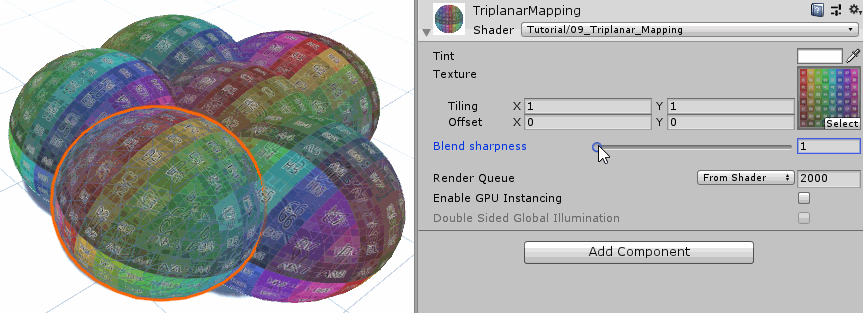Triplanar Mapping still isn’t perfect, it needs tiling textures to work, it breaks at surfaces that are exactly 45° and it’s obviously more expensive than a single texture sample (though not by that much).

You can use it in surface shaders for albedo, specular, etc. maps, but it doesn’t work perfectly for normalmaps without some changes I won’t go into here.

``````Shader "Tutorial/010_Triplanar_Mapping"{
//show values to edit in inspector
Properties{
_Color ("Tint", Color) = (0, 0, 0, 1)
_MainTex ("Texture", 2D) = "white" {}
_Sharpness ("Blend sharpness", Range(1, 64)) = 1
}

//the material is completely non-transparent and is rendered at the same time as the other opaque geometry
Tags{ "RenderType"="Opaque" "Queue"="Geometry"}

Pass{
CGPROGRAM

#include "UnityCG.cginc"

#pragma vertex vert
#pragma fragment frag

//texture and transforms of the texture
sampler2D _MainTex;
float4 _MainTex_ST;

fixed4 _Color;
float _Sharpness;

struct appdata{
float4 vertex : POSITION;
float3 normal : NORMAL;
};

struct v2f{
float4 position : SV_POSITION;
float3 worldPos : TEXCOORD0;
float3 normal : NORMAL;
};

v2f vert(appdata v){
v2f o;
//calculate the position in clip space to render the object
o.position = UnityObjectToClipPos(v.vertex);
//calculate world position of vertex
float4 worldPos = mul(unity_ObjectToWorld, v.vertex);
o.worldPos = worldPos.xyz;
//calculate world normal
float3 worldNormal = mul(v.normal, (float3x3)unity_WorldToObject);
o.normal = normalize(worldNormal);
return o;
}

fixed4 frag(v2f i) : SV_TARGET{
//calculate UV coordinates for three projections
float2 uv_front = TRANSFORM_TEX(i.worldPos.xy, _MainTex);
float2 uv_side = TRANSFORM_TEX(i.worldPos.zy, _MainTex);
float2 uv_top = TRANSFORM_TEX(i.worldPos.xz, _MainTex);

//read texture at uv position of the three projections
fixed4 col_front = tex2D(_MainTex, uv_front);
fixed4 col_side = tex2D(_MainTex, uv_side);
fixed4 col_top = tex2D(_MainTex, uv_top);

//generate weights from world normals
float3 weights = i.normal;
//show texture on both sides of the object (positive and negative)
weights = abs(weights);
//make the transition sharper
weights = pow(weights, _Sharpness);
//make it so the sum of all components is 1
weights = weights / (weights.x + weights.y + weights.z);

//combine weights with projected colors
col_front *= weights.z;
col_side *= weights.x;
col_top *= weights.y;

//combine the projected colors
fixed4 col = col_front + col_side + col_top;

//multiply texture color with tint color
col *= _Color;

return col;
}

ENDCG
}
}